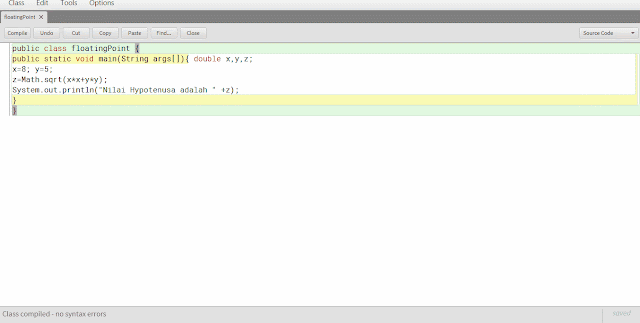Data types JAVA

Data types

Java has two categories of data types, namely object-oriented data types and simple. Object-oriented data types defined by the class, while simple is the basic data types that are owned by as many as eight Java simple data types, known as primitive data types. The primitive data types are as follows:

 This type of data Information boolean Presenting the true / false value byte 8-bit integer char Character double Double-precision floating-point float Single-precision floating-point int Integer (bil. Round) long Long Integer short Short integer

Data Type Integer

Java defines integer data types consisting of byte, short, int and long with each range of values ​​are the following:

 Type width bits The range of values byte 8 -128 to 127 short 16 -32.768 to 32,767 int 32 -2,147,483,648 to 2,147,483,647 long 64 -9,223,372,036,854,775,808 to 9,223,372,036,854,775,807

Integer Coding Examples JAVA:
 `1` `public ``class` `DataInteger {`
 `2` `public static void main(String[] args){ ``long` `ci,im;`
 `3` `im``=``5280` `*` `12``;`
 `4` `ci``=``im``*``im``*``im;`
 `5` `System.out.println(``"Ada "``+``ci``+``" kubik inchi dalam mil kubik."``);`
 `6` `}`
 `7` `}`

Floating-Point Data Types

This data type in Java consists of float and double, each having a different bit width, the float (32 bit) and double (64-bit). Generally the double data type is often used by these functions in Java.

Examples of Floating-Point Coding JAVA:
 `1` `public ``class` `floatingPoint {`
 `2` `public static void main(String args[]){ double x,y,z;`
 `3` `x``=``8``; y``=``5``;`
 `4` `z``=``Math.sqrt(x``*``x``+``y``*``y);`
 `5` `System.out.println(``"Nilai Hypotenusa adalah "` `+``z);`
 `6` `}`
 `7` `}`coding results of floating-point data types

Character Data Types

Unlike programming languages ​​other data type char with a width of 8-bit, but Java uses Unicode (characters used by many languages ​​in the world, for example arabic, starch, etc.) for each character of his, so the bit width of each character is 16 -bit.

Character Coding Examples JAVA:
 `1` `public ``class` `ContohChar {`
 `2` `public static void main(String[] args){ char c;`
 `3` `c``=``'H'``;`
 `4` `System.out.println(``"C berisi "``+``c); c``+``+``;`
 `5` `System.out.println(``"Sekarang C berisi "``+``c); c``=``89``;`
 `6` `System.out.println(``"C berisi "``+``c);`
 `7` `}`
 `8` `}`java coding results with data type character

Boolean Data Types

Boolean type presents a true / false value. JAVA Coding examples for the use of boolean type is as follows:
 `01` `public ``class` `ContohBoolean {`
 `02` `public static void main(String[] args){ boolean b;`
 `03` `b``=``true;`
 `04` `System.out.println(``"b bernilai "``+``b); b``=``false;`
 `05` `System.out.println(``"b bernilai "``+``b);`
 `06` `if``(b)`
 `07` `System.out.println(``"b pasti bernilai true"``);`
 `08` `else`
 `09` `System.out.println(``"b pasti bernilai false"``);`
 `10` `System.out.println(``"7 < 9 adalah "``+``(``7` `< ``9``));`
 `11` `}`
 `12` `}`

Literals (constants)

Literal refers to a fixed rate that is served and can be understood by humans, such as the 100 or the character 'b' (enclosed in quotation marks, while a string enclosed in quotes). By default writing numbers in Java is an integer, but if you want a long type, use the literal L at the end of the number, eg 12L (12 long integer value).

Writing hex numbers start with 0x, for example 0x2F. For octal numbers begin with the number 0, for example 023.

Characters circuit Escape

For special characters outside of the standard characters, for example the character Carriage Return, Tab, Line Feed and forth using the symbol '\'.

 Escape circuit Information \ ' single quote \ " Double quote \\ backslash \ r carriage return \ n New line \ f Form feed \ t horizontal tabs \ b Backspace \ ddd Octal constant (where ddd is an octal constant) \ uxxxx Hexadecimal constant (where xxxx is a hexadecimal constant)

string Literal

Value strings in Java flanked by using quotation (quotation).
Coding example JAVA String Literal:
 `1` `public ``class` `ContohStringLiteral {`
 `2` `public static void main(String[] args){`
 `3` `System.out.``print``(``"Ini merupakan baris pertama, kemudian disusul dengan \n baris kedua!\n"``);`
 `4` `System.out.``print``(``"Contoh penggunaan \"backslash\" '\\'.\n"``); System.out.``print``(``"Tabulasi Tab1\tTab2\tTab3"``);`
 `5` `}`
 `6` `}`coding results with java string literals
Artikel Terkait: